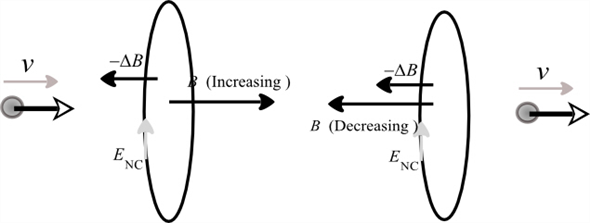Problem

# If magnetic monopoles existed, the right-hand side of Eq. (29.20) would include a term p...

If magnetic monopoles existed, the right-hand side of Eq. (29.20) would include a term proportional to the current of magnetic monopoles. Suppose a steady monopole current is moving in a long straight wire. Sketch the electric field lines that such a current would produce.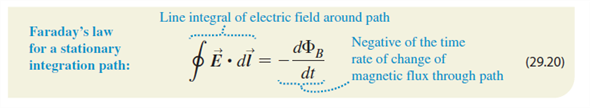#### Step-by-Step Solution

Solution 1

Solution:

If the monopole approaches the loop, the magnetic field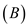associated with the coil increases, the rate of change of magnetic field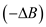associated with the coil will be directed towards left. If this change in magnetic field is in the right thumb direction, then the remaining fingers which curl around represent the non-columbic electric field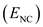direction. If the monopole reseeds from the loop, the magnetic fieldassociated with the coil decreases, the rate of change of magnetic fieldassociated with the coil will be directed towards left. If this change in magnetic field is in the right thumb direction, then the remaining fingers which curl around represent the non-columbic electric fielddirection. The schematic diagram for both the cases is shown in the below figure.